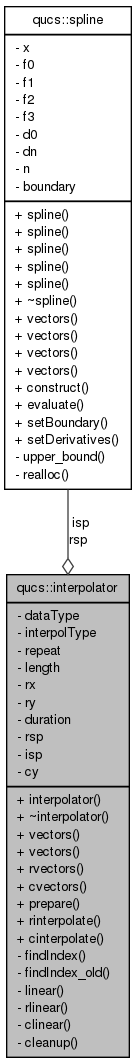Qucs-core  0.0.19
qucs::interpolator Class Reference

`#include <interpolator.h>`

Collaboration diagram for qucs::interpolator:[legend]

## Public Member Functions

interpolator ()
~interpolator ()
void vectors (nr_double_t *, nr_double_t *, int)
void vectors (nr_complex_t *, nr_double_t *, int)
void rvectors (qucs::vector *, qucs::vector *)
void cvectors (qucs::vector *, qucs::vector *)
void prepare (int, int, int domain=DATA_RECTANGULAR)
nr_double_t rinterpolate (nr_double_t)
nr_complex_t cinterpolate (nr_double_t)

## Private Member Functions

int findIndex (nr_double_t)
int findIndex_old (nr_double_t)
nr_double_t linear (nr_double_t, nr_double_t, nr_double_t, nr_double_t, nr_double_t)
nr_double_t rlinear (nr_double_t, int)
nr_complex_t clinear (nr_double_t, int)
void cleanup (void)

## Private Attributes

int dataType
int interpolType
int repeat
int length
nr_double_trx
nr_double_try
nr_double_t duration
splinersp
splineisp
nr_complex_tcy

## Detailed Description

Definition at line 45 of file interpolator.h.

## Constructor & Destructor Documentation

 qucs::interpolator::interpolator ( )

Definition at line 43 of file interpolator.cpp.

 qucs::interpolator::~interpolator ( )

Definition at line 53 of file interpolator.cpp.

## Member Function Documentation

 nr_complex_t qucs::interpolator::cinterpolate ( nr_double_t x )

Definition at line 315 of file interpolator.cpp.

 void qucs::interpolator::cleanup ( void ) ` [private]`

Definition at line 62 of file interpolator.cpp.

 nr_complex_t qucs::interpolator::clinear ( nr_double_t x, int idx ) ` [private]`

Definition at line 258 of file interpolator.cpp.

 void qucs::interpolator::cvectors ( qucs::vector * y, qucs::vector * x )

Definition at line 123 of file interpolator.cpp.

 int qucs::interpolator::findIndex ( nr_double_t x ) ` [private]`

Definition at line 211 of file interpolator.cpp.

 int qucs::interpolator::findIndex_old ( nr_double_t x ) ` [private]`

Definition at line 234 of file interpolator.cpp.

 nr_double_t qucs::interpolator::linear ( nr_double_t x, nr_double_t x1, nr_double_t x2, nr_double_t y1, nr_double_t y2 ) ` [private]`

Definition at line 243 of file interpolator.cpp.

 void qucs::interpolator::prepare ( int interpol, int repitition, int domain = `DATA_RECTANGULAR` )

Definition at line 142 of file interpolator.cpp.

 nr_double_t qucs::interpolator::rinterpolate ( nr_double_t x )

Definition at line 271 of file interpolator.cpp.

 nr_double_t qucs::interpolator::rlinear ( nr_double_t x, int idx ) ` [private]`

Definition at line 253 of file interpolator.cpp.

 void qucs::interpolator::rvectors ( qucs::vector * y, qucs::vector * x )

Definition at line 86 of file interpolator.cpp.

 void qucs::interpolator::vectors ( nr_double_t * y, nr_double_t * x, int len )

Definition at line 69 of file interpolator.cpp.

 void qucs::interpolator::vectors ( nr_complex_t * y, nr_double_t * x, int len )

Definition at line 105 of file interpolator.cpp.

## Field Documentation

 nr_complex_t* qucs::interpolator::cy` [private]`

Definition at line 77 of file interpolator.h.

 int qucs::interpolator::dataType` [private]`

Definition at line 69 of file interpolator.h.

 nr_double_t qucs::interpolator::duration` [private]`

Definition at line 75 of file interpolator.h.

 int qucs::interpolator::interpolType` [private]`

Definition at line 70 of file interpolator.h.

 spline * qucs::interpolator::isp` [private]`

Definition at line 76 of file interpolator.h.

 int qucs::interpolator::length` [private]`

Definition at line 72 of file interpolator.h.

 int qucs::interpolator::repeat` [private]`

Definition at line 71 of file interpolator.h.

 spline* qucs::interpolator::rsp` [private]`

Definition at line 76 of file interpolator.h.

 nr_double_t* qucs::interpolator::rx` [private]`

Definition at line 73 of file interpolator.h.

 nr_double_t* qucs::interpolator::ry` [private]`

Definition at line 74 of file interpolator.h.

The documentation for this class was generated from the following files: